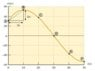# Velocity vs. time graph

computing
Member warned that some effort must be shown
Homework Statement:
v-t graphing
Relevant Equations:
no equation
hi
how can i draw velocity - time graph to determine whetere this particle has ever exceeeded the 30 km/h velocity or not ?

#### Attachments

•physics forum.jpg
14.5 KB · Views: 156

archaic
If that is what you are asked to do, you can draw the average velocity over each ##\Delta t##, just use the given formula.

Staff Emeritus
Homework Statement:: v-t graphing
Homework Equations:: no equation

hi
how can i draw velocity - time graph to determine whetere this particle has ever exceeeded the 30 km/h velocity or not ?

First of all, let's check if you have the conceptual understanding of the relationship between displacement-time graph, and velocity-time graph. Do you know the relationship between those two graphs? Or more importantly, do you know how to find the velocity if you are given a displacement over a time period?

The graph gives you a hint on what you need to get a rough estimate of the velocity, i.e. it gives you a measure of the displacement Δx and the time for that displacement Δt.

Zz.

•